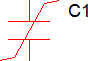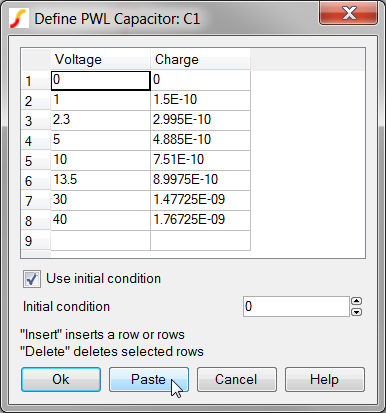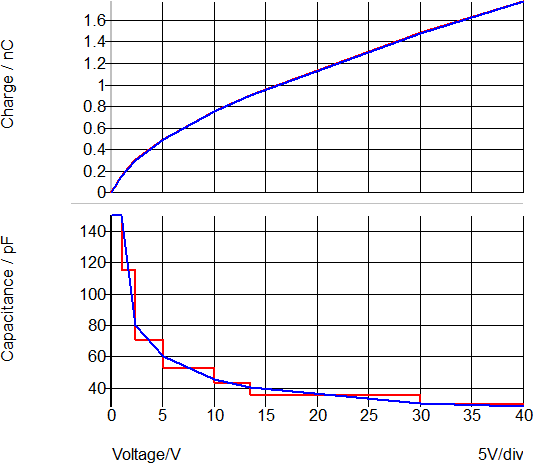# PWL Capacitors

The SIMPLIS Piecewise Linear (PWL) capacitor is used to model a voltage-dependent capacitance, such as a junction capacitance. The PWL capacitor is defined with a series of points on an x-y plane where the charge is on the vertical axis and the voltage is on the horizontal axis. With this definition, the charge is always continuous as the voltage across the capacitor varies.A common use for the PWL capacitor is to model the non-linear capacitances in semiconductors. The model parameter extraction routines automatically generate the points to define SIMPLIS PWL capacitors.

A good description of the PWL modeling techniques using PWL capacitors can be found in the Piecewise Linear Capacitors section in SIMPLIS is Always Piecewise Linear, All the Time.

In this topic:

## Circuit Example

This circuit example uses a spreadsheet to curve fit a non-linear junction capacitance to a PWL model. The spreadsheet and the circuit file can be downloaded here: simplis_004_pwl_capacitor_example.zip

The curve fit procedure is as follows:

1. The data sheet curve was approximated in the spreadsheet.
2. PWL points were then calculated from the data sheet curve.
3. The spreadsheet cells defining the points (I2 through J11)  were selected, and copied to the windows clipboard.
4. On the Define PWL Capacitor dialog, the copied cells were pasted using the Paste button.## Waveforms

The simulation compares the PWL capacitor charge and capacitance to the curves extracted from the datasheet.

• The capacitance is on the lower graph.
• The red curve is the PWL capacitor
• The blue curve is the datasheet capacitance curve.
Important: Notice the capacitance "steps" in the SIMPLIS PWL model.
• The upper curve is the integral of the capacitance; that is, the charge.
Important: Notice what may not, at first, be intuitive: the PWL model closely matches the actual charge of the non-linear capacitor, despite the PWL steps in capacitance.